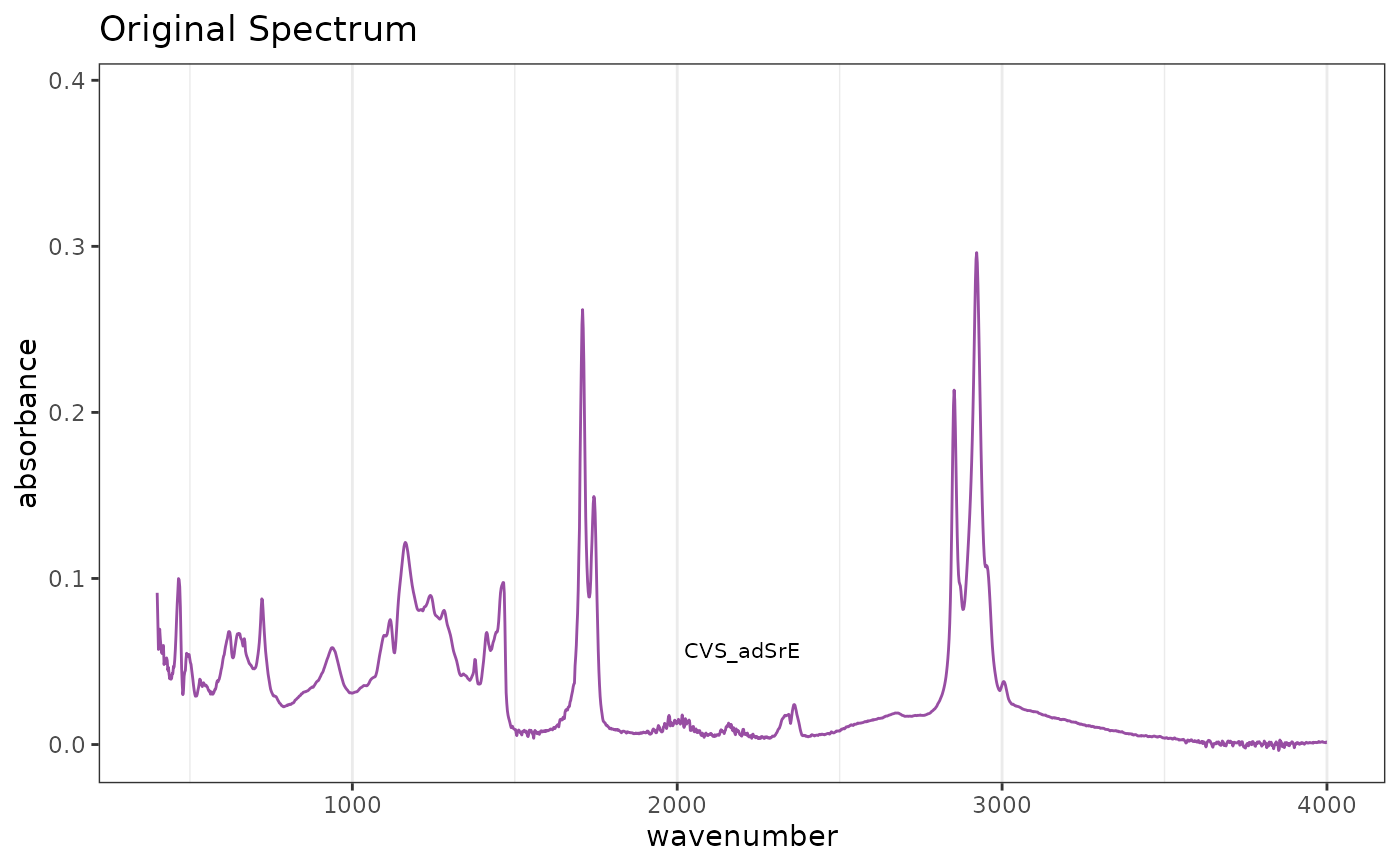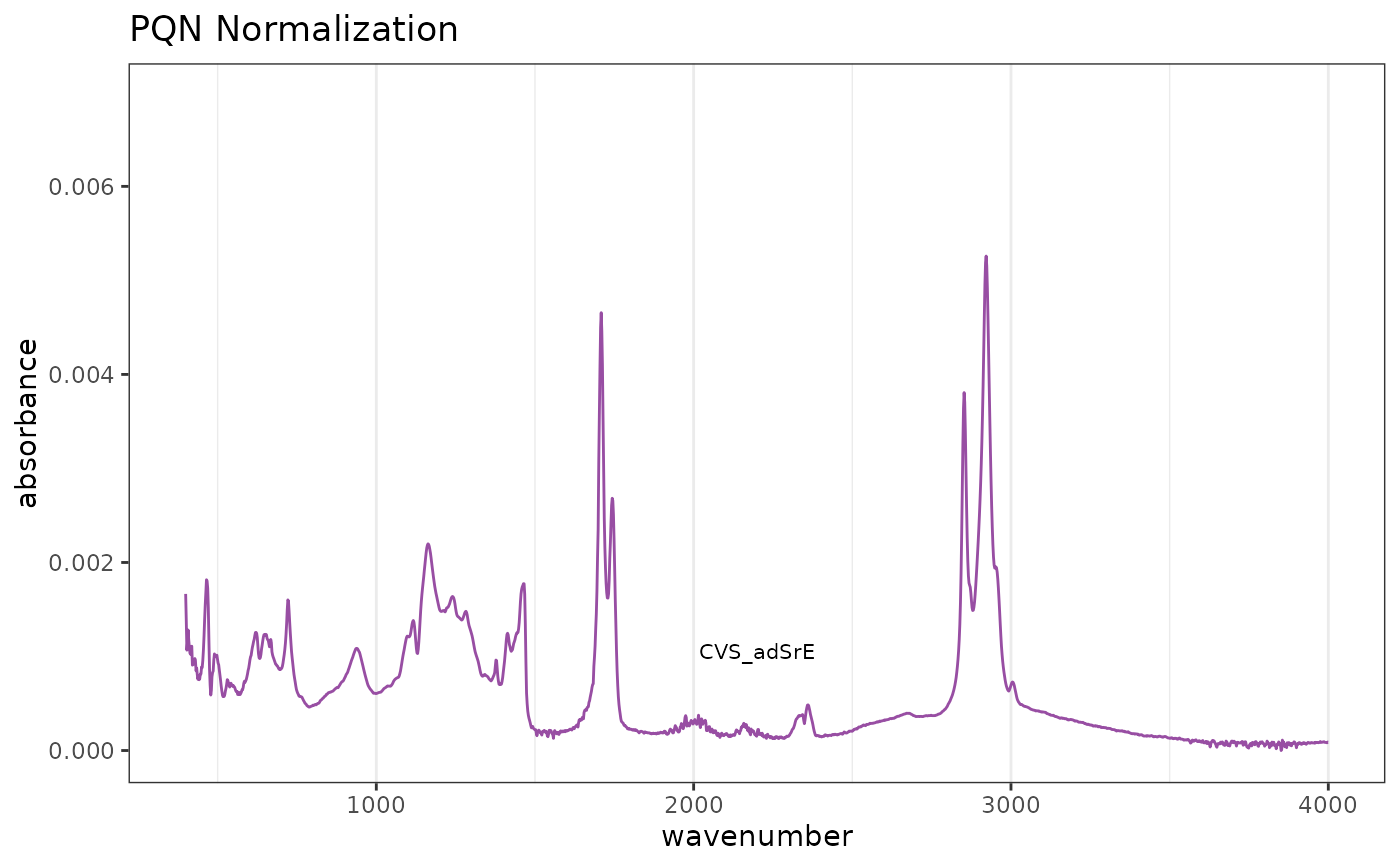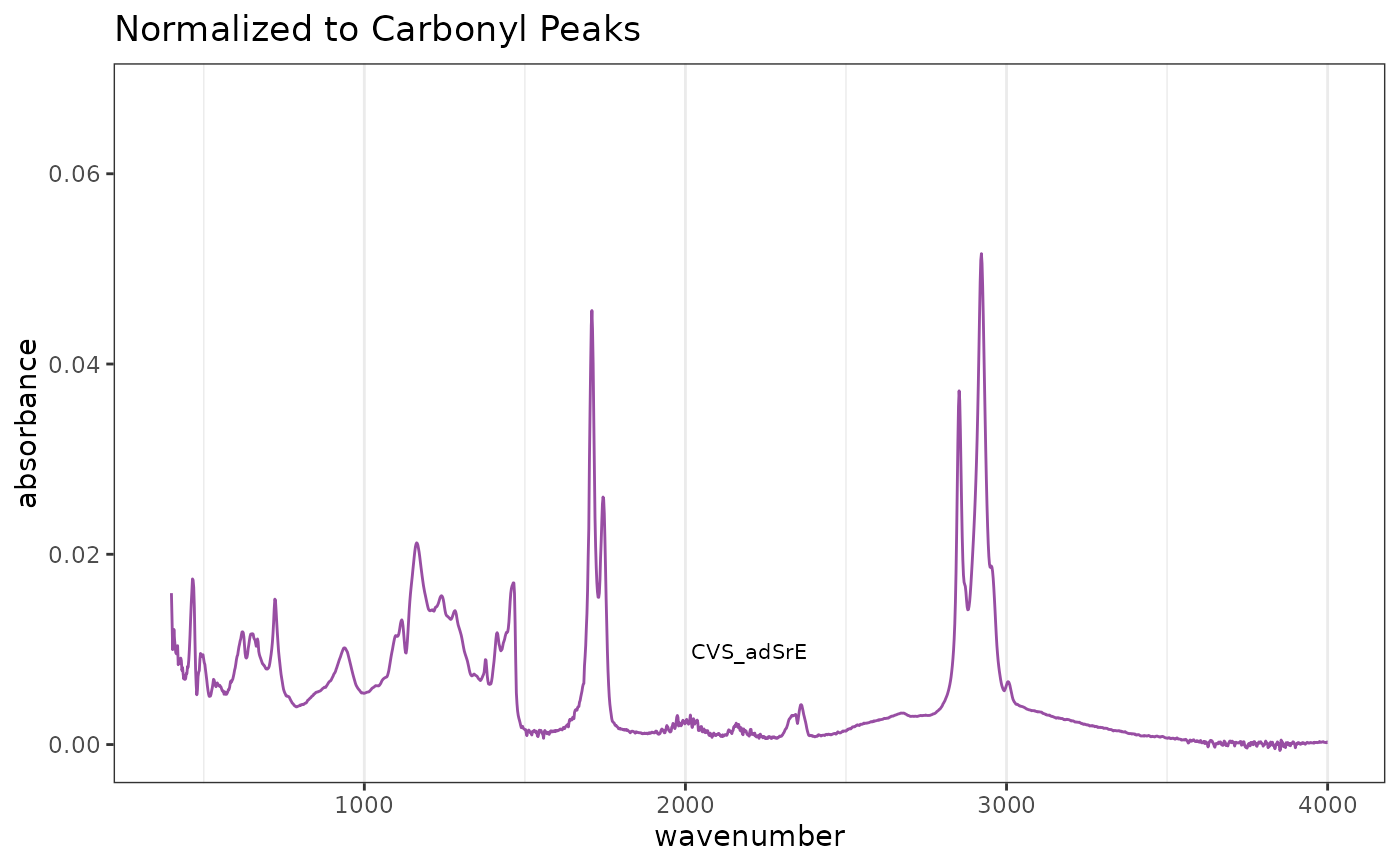This function carries out normalization of the spectra in a Spectra object. There are currently four options:

• "PQN" carries out "Probabalistic Quotient Normalization" as described in the reference. This is probably the best option for many data sets. However, please be careful if your sample has protein in it, PQN is potentially biased. See the references.

• "TotInt" normalizes by total intensity. In this case, the y-data of a Spectra object is normalized by dividing each y-value by the sum of the y-values in a given spectrum. Thus each spectrum sums to 1. This method assumes that the total concentration of all substances giving peaks does not vary across samples which may not be true.

• "Range" allows one to do something similar to "TotInt" but rather than using the sum of the entire spectrum as the denominator, only the sum of the given range is used. This would be appropriate if there was an internal standard in the spectrum which was free of interferance, and one wanted to normalize relative to it.

• "zero2one" scales each spectrum separately to a [0...1] scale. This is sometimes useful for visual comparison of chromatograms but is inappropriate for spectral data sets.

normSpectra(spectra, method = "PQN", RangeExpress = NULL)

## Arguments

spectra

An object of S3 class Spectra().

method

One of c("PQN", "TotInt", "Range", "zero2one") giving the method for normalization.

RangeExpress

A vector of logicals (must be of length(Spectra$freq)). This vector should be TRUE for the frequency range you want to serve as the basis for norming, and FALSE otherwise. The entire spectrum will be divided by the sum of the TRUE range. See the examples. ## Value An object of S3 class Spectra(). ## See also Additional documentation at https://bryanhanson.github.io/ChemoSpec/ ## Author Bryan A. Hanson (DePauw University). ## Examples # This example assumes the graphics output is set to ggplot2 (see ?GraphicsOptions). library("ggplot2") data(SrE.IR) # Reference spectrum before normalization p1 <- plotSpectra(SrE.IR) + ggtitle("Original Spectrum") p1# Default PQN normalization res1 <- normSpectra(SrE.IR) p2 <- plotSpectra(res1) + ggtitle("PQN Normalization") p2# Norm over carbonyl region RE <- SrE.IR$freq > 1650 & SrE.IR$freq < 1800 res2 <- normSpectra(SrE.IR, method = "Range", RangeExpress = RE) p3 <- plotSpectra(res2) + ggtitle("Normalized to Carbonyl Peaks") p3# Check numerically rowSums(res2$data[, RE]) # compare to rowSums(SrE.IR\$data[,RE])
#>   1 1 1 1 1 1 1 1 1 1 1 1 1 1 1 1